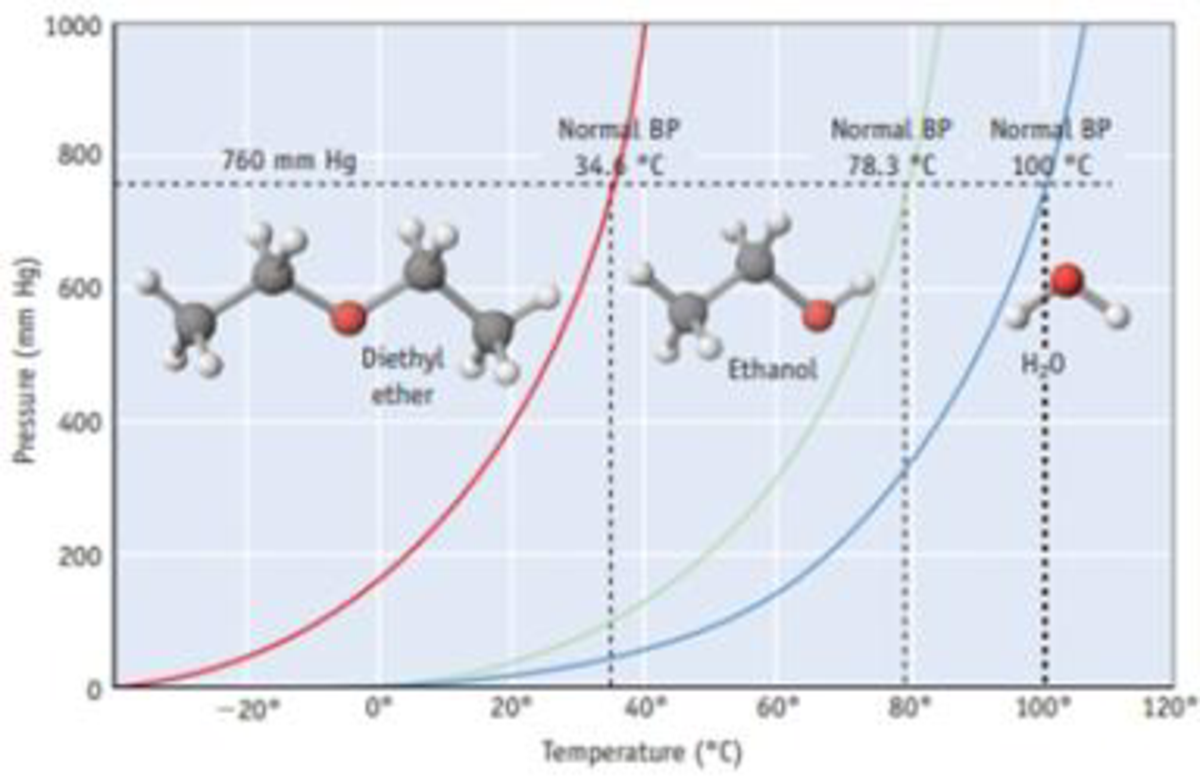# Answer the following questions using Figure 11.12: (a) What is the equilibrium vapor pressure of diethyl ether at room temperature (approximately 20 °C)? (b) Place the three compounds in Figure 11.12 in order of increasing intermolecular forces. (c) If the pressure in a flask is 400 mm Hg and if the temperature is 40 °C, which of the three compounds (diethyl ether, ethanol, and water) are liquids, and which are gases? Figure 11.12 Vapor pressure curves for diethyl ether [(C 2 H 3 ) 2 O], ethanol (C 2 H 5 OH), and water. Each curve represents conditions of T and P of which the two phases, liquid and vapor, are in equilibrium. These compounds exist as liquids for temperatures and pressures to the left of the curve and as gases under conditions to the right of the curve. (See Appendix G for vapor pressures for water of various temperatures.)### Chemistry & Chemical Reactivity

9th Edition
John C. Kotz + 3 others
Publisher: Cengage Learning
ISBN: 9781133949640

#### Solutions

Chapter
Section### Chemistry & Chemical Reactivity

9th Edition
John C. Kotz + 3 others
Publisher: Cengage Learning
ISBN: 9781133949640
Chapter 11, Problem 14PS
Textbook Problem
217 views

## Answer the following questions using Figure 11.12: (a) What is the equilibrium vapor pressure of diethyl ether at room temperature (approximately 20 °C)? (b) Place the three compounds in Figure 11.12 in order of increasing intermolecular forces. (c) If the pressure in a flask is 400 mm Hg and if the temperature is 40 °C, which of the three compounds (diethyl ether, ethanol, and water) are liquids, and which are gases?Figure 11.12 Vapor pressure curves for diethyl ether [(C2H3)2O], ethanol (C2H5OH), and water. Each curve represents conditions of T and P of which the two phases, liquid and vapor, are in equilibrium. These compounds exist as liquids for temperatures and pressures to the left of the curve and as gases under conditions to the right of the curve. (See Appendix G for vapor pressures for water of various temperatures.)

(a)

Interpretation Introduction

Interpretation: The equilibrium vapor pressure of dimethyl ether at room temperature should be determined.

Concept Introduction: Vapor pressure is nothing but the pressure of a vapor in contact with its liquid or solid form.

When a liquid and vapor are in equilibrium the pressure exerted by the vapor is called the equilibrium vapor pressure.

### Explanation of Solution

The figure is given,

(b)

Interpretation Introduction

Interpretation: The increasing order of intermolecular forces of the given three compounds has to be determined.

Concept Introduction: Vapor pressure is nothing but the pressure of a vapor in contact with its liquid or solid form.

When a liquid and vapor are in equilibrium the pressure exerted by the vapor is called the equilibrium vapor pressure.

If intermolecular force is small the vapor pressure of the substance is high and the boiling point will be low.

(c)

Interpretation Introduction

Interpretation: The compounds that are in liquid and in gaseous state when the equilibrium vapor pressure is 400mmHg and at temperature 400C should be determined.

Concept Introduction: Vapor pressure is nothing but the pressure of a vapor in contact with its liquid or solid form.

When a liquid and vapor are in equilibrium the pressure exerted by the vapor is called the equilibrium vapor pressure.

If intermolecular force is small the vapor pressure of the substance is high and the boiling point will be low.

### Still sussing out bartleby?

Check out a sample textbook solution.

See a sample solution

#### The Solution to Your Study Problems

Bartleby provides explanations to thousands of textbook problems written by our experts, many with advanced degrees!

Get Started

Find more solutions based on key concepts
The following are complementary proteins: pot roast and chicken pot roast and carrots rice and French fries pea...

Nutrition: Concepts and Controversies - Standalone book (MindTap Course List)

Define the following terms: a. chromosome b. chromatin

Human Heredity: Principles and Issues (MindTap Course List)

Match the term listed in Column A with its definition from Column B

Nutrition Through the Life Cycle (MindTap Course List)

How does an arthropod growth curve compare with yours?

Oceanography: An Invitation To Marine Science, Loose-leaf Versin

Review. A standing-wave pattern is set up by radio waves between two metal sheets 2.00 m apart, which is the sh...

Physics for Scientists and Engineers, Technology Update (No access codes included)